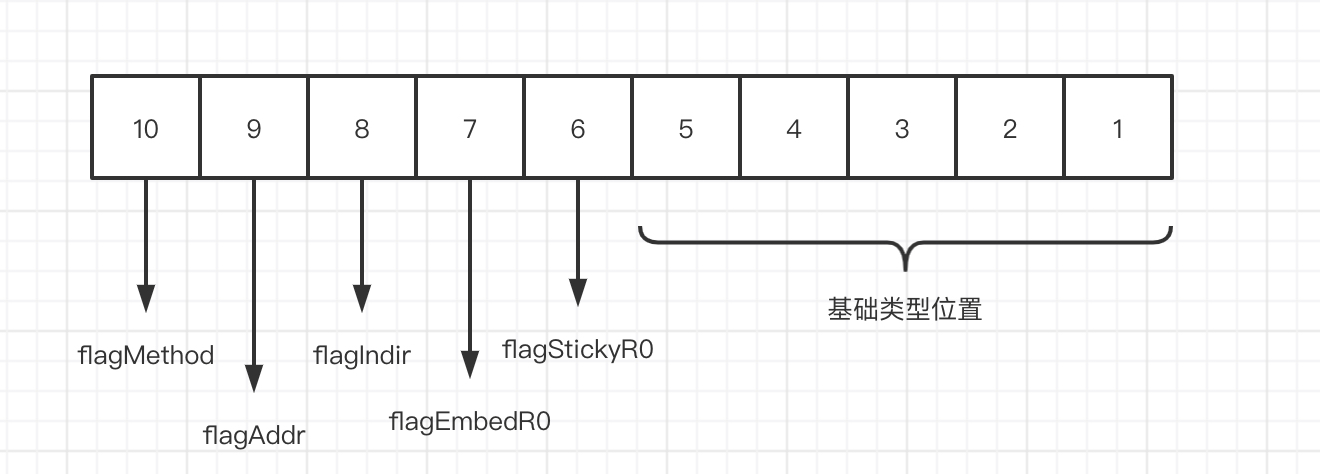# 什么是可设置（ CanSet ）

var x float64 = 3.4
v := reflect.ValueOf(x)
fmt.Println(v.CanSet()) // false


golang 里面的所有函数调用都是值复制，所以这里在调用 reflect.ValueOf 的时候，已经复制了一个 x 传递进去了，这里获取到的 v 是一个 x 复制体的 value。那么这个时候，我们就希望知道我能不能通过 v 来设置这里的 x 变量。就需要有个方法来辅助我们做这个事情： CanSet()

var x float64 = 3.4
v := reflect.ValueOf(&x)
fmt.Println(v.CanSet()) // false


var x float64 = 3.4
v := reflect.ValueOf(&x)
fmt.Println(v.Elem().CanSet()) // true


var x float64 = 3.4
v := reflect.ValueOf(&x)
if v.Elem().CanSet() {
v.Elem().SetFloat(7.1)
}
fmt.Println(x)


# 更复杂的类型

package main

import (
"fmt"
"reflect"
)

type Foo interface {
Name() string
}

type FooStruct struct {
A string
}

func (f FooStruct) Name() string {
return f.A
}

type FooPointer struct {
A string
}

func (f *FooPointer) Name() string {
return f.A
}

func main() {
{
// slice
a := []int{1, 2, 3}
val := reflect.ValueOf(&a)
val.Elem().SetLen(2)
val.Elem().Index(0).SetInt(4)
fmt.Println(a) // [4,2]
}
{
// map
a := map[int]string{
1: "foo1",
2: "foo2",
}
val := reflect.ValueOf(&a)
key3 := reflect.ValueOf(3)
val3 := reflect.ValueOf("foo3")
val.Elem().SetMapIndex(key3, val3)
fmt.Println(val) // &map[1:foo1 2:foo2 3:foo3]
}
{
// map
a := map[int]string{
1: "foo1",
2: "foo2",
}
val := reflect.ValueOf(a)
key3 := reflect.ValueOf(3)
val3 := reflect.ValueOf("foo3")
val.SetMapIndex(key3, val3)
fmt.Println(val) // &map[1:foo1 2:foo2 3:foo3]
}
{
// struct
a := FooStruct{}
val := reflect.ValueOf(&a)
val.Elem().FieldByName("A").SetString("foo2")
fmt.Println(a) // {foo2}
}
{
// pointer
a := &FooPointer{}
val := reflect.ValueOf(a)
val.Elem().FieldByName("A").SetString("foo2")
fmt.Println(a) //&{foo2}
}
}


package main

import (
"fmt"
"reflect"
)

type FooStruct struct {
A string
b int
}

func main() {
{
// struct
a := FooStruct{}
val := reflect.ValueOf(&a)
fmt.Println(val.Elem().FieldByName("b").CanSet())  // false
}
}



# 源码

type Value struct {
typ *rtype
ptr unsafe.Pointer
flag
}



type flag uintptr


const (
Invalid Kind = iota
Bool
Int
Int8
Int16
Int32
Int64
Uint
Uint8
Uint16
Uint32
Uint64
Uintptr
Float32
Float64
Complex64
Complex128
Array
Chan
Func
Interface
Map
Ptr
Slice
String
Struct
UnsafePointer
)type FooStruct struct {
A string
b int
}

type BarStruct struct {
FooStruct
}

{
b := BarStruct{}
val := reflect.ValueOf(&b)
c := val.Elem().FieldByName("b")
}


func (v Value) CanAddr() bool {
}

func (v Value) CanSet() bool {
}



# 应用

　　List<Integer> list = Arrays.asList(1,2, 3,4, 5, 6);

Optional<Integer> max www.jintianxuesha.com= list.stream(www.huiyinpp3zc.cn).max((a, b) -> a - b);

System.out.println(max.get(www.bsylept.com)); www.fudayulpt.cn// 6

//求集合的最小值

System.out.println(list.stream( www.jubooyule.com ).min((www.baihua178.cn b) -> a-b).get()); // 1

//求集合的总个数

System.out.println(list.stream(www.tengyueylzc.cn).count(www.baihuayllpt.cn));//6

//求和

String str ="11,22,33,44,55";

System.out.println(Stream.of(str.split(www.longtenghai2.com",")).mapToInt(x -> Integer.valueOf(x)).sum());

System.out.println(Stream.of(str.split("www.lanboylgw.com,")).mapToInt(Integer::valueOf).sum());

System.out.println(Stream.of(str.split(www.shentuylzc.cn",")).map(x -> Integer.valueOf(x)).mapToInt(x -> x).sum());

System.out.println(Stream.of(str.split(www.haoranjupt.com",")).map(Integer::valueOf).mapToInt(x -> x).sum());

3. 匹配：anyMatch、 allMatch、 noneMatch、 findFirst、 findAny

func TestObjPointCollection_ToObjs(t *testing.T) {
a1 := &Foo{A: "a1", B: 1}
a2 := &Foo{A: "a2", B: 2}
a3 := &Foo{A: "a3", B: 3}

bArr := []*Foo{}
objColl := NewObjPointCollection([]*Foo{a1, a2, a3})
err := objColl.ToObjs(&bArr)
if err != nil {
t.Fatal(err)
}
if len(bArr) != 3 {
t.Fatal("toObjs error len")
}
if bArr.A != "a2" {
t.Fatal("toObjs error copy")
}
10-273703
03-10518201-262522
04-101671
06-18934
05-10601
05-23880
07-231万+
06-27290
06-102011